2Motion of a charged particle in the magnetic field
Оценка 4.7

# 2Motion of a charged particle in the magnetic field

Оценка 4.7
docx
07.05.2020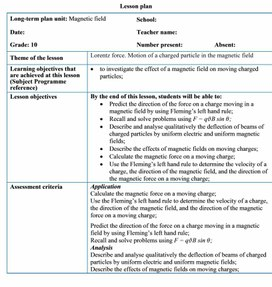2Motion of a charged particle in the magnetic field.docx

Lesson plan

Long-term plan unit: Magnetic field

School:

Date:

Teacher name:

Number present:

Absent:

Theme of the lesson

Lorentz force. Motion of a charged particle in the magnetic field

Learning objectives that are achieved at this lesson (Subject Programme reference)

·         to investigate the effect of a magnetic field on moving charged particles;

Lesson objectives

By the end of this lesson, students will be able to:

·         Predict the direction of the force on a charge moving in a magnetic field by using Fleming’s left hand rule;

·     Recall and solve problems using F = q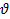B sin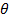;

·         Describe and analyse qualitatively the deflection of beams of charged particles by uniform electric and uniform magnetic fields;

·         Describe the effects of magnetic fields on moving charges;

·         Calculate the magnetic force on a moving charge;

·         Use the Fleming’s left hand rule to determine the velocity of a charge, the direction of the magnetic field, and the direction of the magnetic force on a moving charge;

Assessment criteria

Application

Calculate the magnetic force on a moving charge;

Use the Fleming’s left hand rule to determine the velocity of a charge, the direction of the magnetic field, and the direction of the magnetic force on a moving charge;

Predict the direction of the force on a charge moving in a magnetic field by using Fleming’s left hand rule;

Recall and solve problems using F = qB sin;

Analysis

Describe and analyse qualitatively the deflection of beams of charged particles by uniform electric and uniform magnetic fields;

Describe the effects of magnetic fields on moving charges;

Language objectives

Subject-specific vocabulary & terminology

magnetic force on a moving charge

a beam of electrons

electron gun

Fleming’s left-hand rule

deflected up the page

deflected down the page

into the page

out of page

the magnetic flux density B (strength of the magnetic field)

the charge Q on the particle

the speed v of the particle

Useful set(s) of phrases for dialogue/writing

A charged particle entering at right angles to a uniform magnetic field describes a circular path because the magnetic force is perpendicular to

the velocity.

Type of differentiation

Differentiated poster-session , Collaborative Learning, Progressive Task with Digital resources

Values instilled at the lesson

Safety, Consideration to others, Co-operation, Opportunity for Life-Long Learning, Academic Integrity and Transparency, Respect for Self and Others

Mathematics: deducing the equation F = q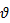B sin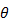;

ICT skills

Research skills, use of video as introduction

Previous learning

Grade 8: magnetic fields; representation of fields by field lines; fields of permanent magnets

Grade 8: electrical equations: V = IR, P = IV

Course of the lesson

Planned stages of the lesson

Planned activities at the lesson

Resources

Beginning

(0-3 min)

(4-6 min)

Teacher:

-Introduces the topic of day and spelling out the learning outcome they will possess after the study.

1. Organizational moment to acquaint students with the

·         The theme of the lesson

·         The objectives of the lesson

·         The criteria of success for the lesson

·         The plan of events for the lesson

# ·         What does “the whole picture” mean?A bubble chamber image of the tracks of subatomic

particles. The tracks curve because the charged

particles are affected by the presence of a magnetic field.

Our lesson today is…….

Middle

7-27 min

(D) Demonstration: Bending an electron beam

If a fine beam electron tube is available, the sight of the paths left by electrons travelling in a circular path when a magnetic field is applied makes a good introduction to this lesson. If the apparatus is not available, then using a picture offers an alternative approach to students to explain.

Electron beam tubes

An electron beam tube (Figure) can be used to demonstrate the magnetic force on a moving charge. A beam of electrons is produced by an ‘electron gun’, and magnets or electromagnets are used to apply a magnetic field.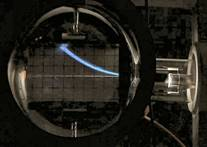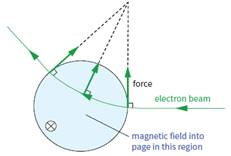The magnetic force on a moving charge

The factors that determine the size of the force on a moving charge in a uniform magnetic field. It will depend on:

■■ the magnetic flux density B (strength of the magnetic field)

■■ the charge Q on the particle

■■ the speed v of the particle.

Discussion: Deducing F = BeV
Any moving charge is an electric current, whether or not the charge is flowing through a material or not. Therefore, it is not unreasonable to expect to find a force on a charged particle moving through space.

Suppose we have such a particle with a charge q, moving at a speed v, at right angles to a magnetic field of flux density B. In a time t, the charge will move a distance L = v∙t and is equivalent to a current I = q / t.

Force on the current

F = BIL = B ∙ q / t ∙ v ∙ t = Bqv

If the field and current are at an angle q, then the formula will be modified to F = Bqv sinϑ

The force F is always at right angles to the particle’s velocity v, and its direction can be found using the Fleming’s left-hand rule.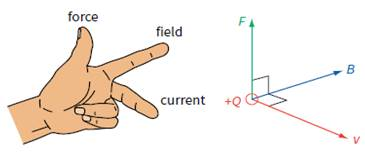28-37 min

(I) Individual work. WHITEBOARD  ACTIVITY

Teacher provides students with a mini whiteboard or a A4 paper and markers. As students know the answer for each question, they write and show it to teacher. Teacher check and assess students ability to use Fleming’s left hand rule to determine the velocity of a charge, the direction of the magnetic field, and the direction of the magnetic force on a moving charge.

1. What is the direction of the magnetic force on a positive charge that moves as shown in each of the six cases shown below: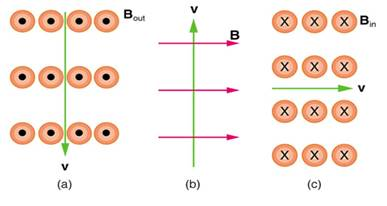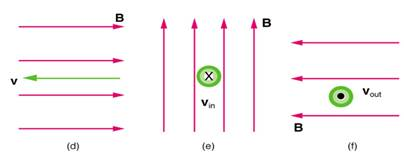2. What is the maximum force on an aluminum rod with a  charge that you pass between the poles of a 1.50 T permanent magnet at a speed of 5.00 m/s? In what direction is the force?

Ending

(38-40 min)

At the end of the lesson, learners reflect on their learning:

-           What has been learned

-           What remained unclear

-           What is necessary to work on

Where possible the learners could evaluate their own work as well as the work of their classmates using certain criteria.

Differentiation – how do you plan to give more support? How do you plan to challenge the more able learners?

Assessment – how are you planning to check students’ learning?

Health and safety regulations

·         Multiple Intelligences

-          Visual will watch the video

-          Analytical take information from the texts

·         Differentiation by questioning and dividing in group

·         Worksheet with varied difficulties

Assessment – how are you planning to check students’ learning?

The output for the worksheet will serve as assessment

Questions during the lesson will also serve as formative assessment.

Be careful when use the laser-coder

Reflection

Were the lesson objectives/learning objectives realistic? Did all learners achieve the LO?

If not, why?

Did my planned differentiation work well?

Did I stick to timings?

What changes did I make from my plan and why?

Use the space below to reflect on your lesson. Answer the most relevant questions from the box on the left about your lesson.

Summary evaluation

What two things went really well (consider both teaching and learning)?

1:

2:

What two things would have improved the lesson (consider both teaching and learning)?

1:

2:

What have I learned from this lesson about the class or achievements/difficulties of individuals that will inform my next lesson?

Скачано с www.znanio.ru

## Language objectives Subject-specific vocabulary & terminology magnetic force on a moving charge a beam of electrons electron gun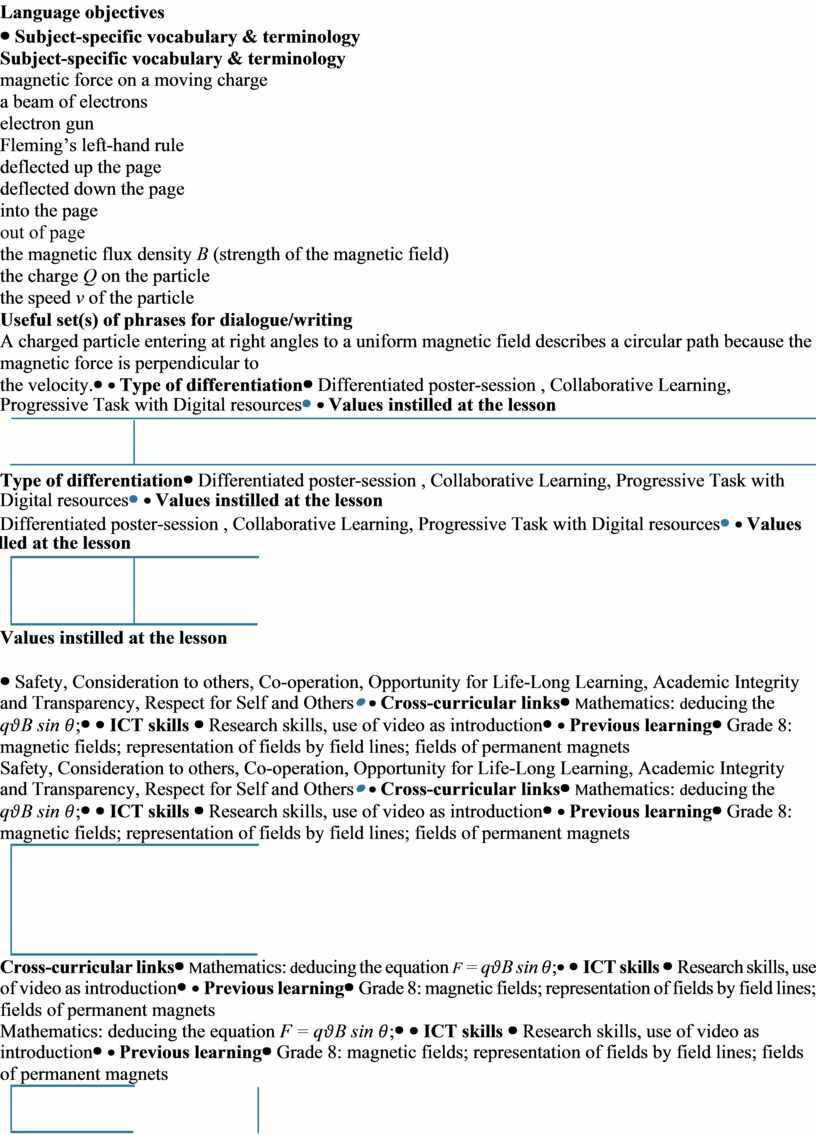## A bubble chamber image of the tracks of subatomic particles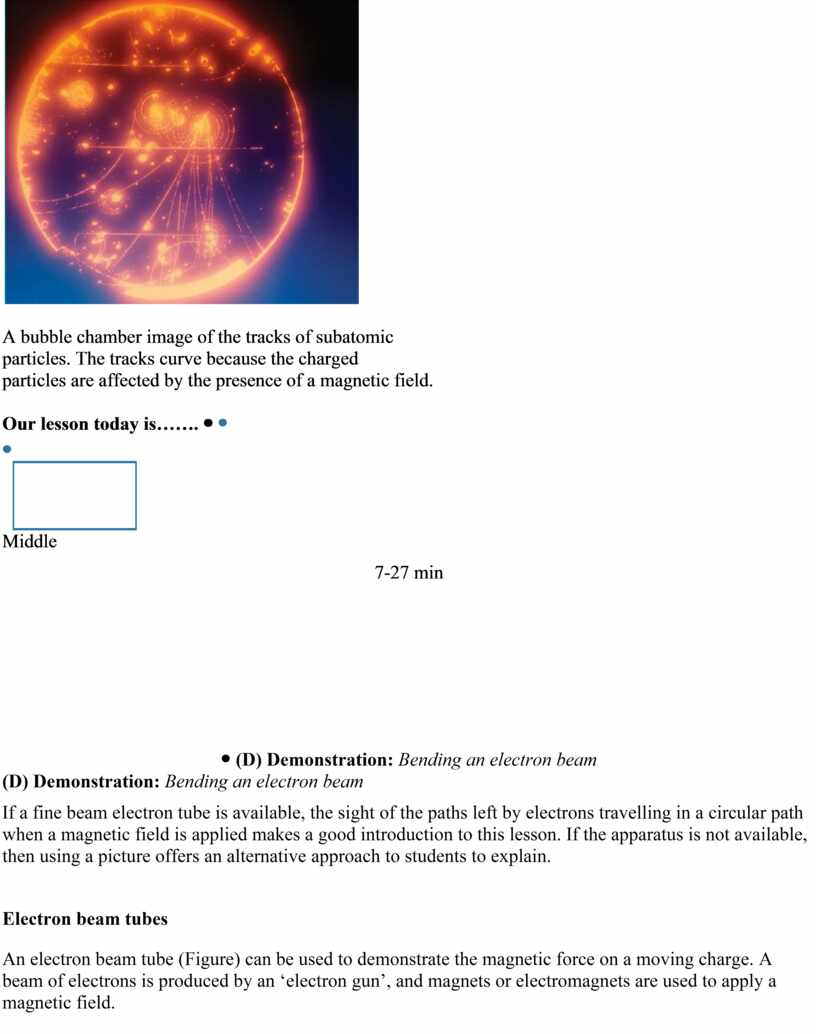## Any moving charge is an electric current, whether or not the charge is flowing through a material or not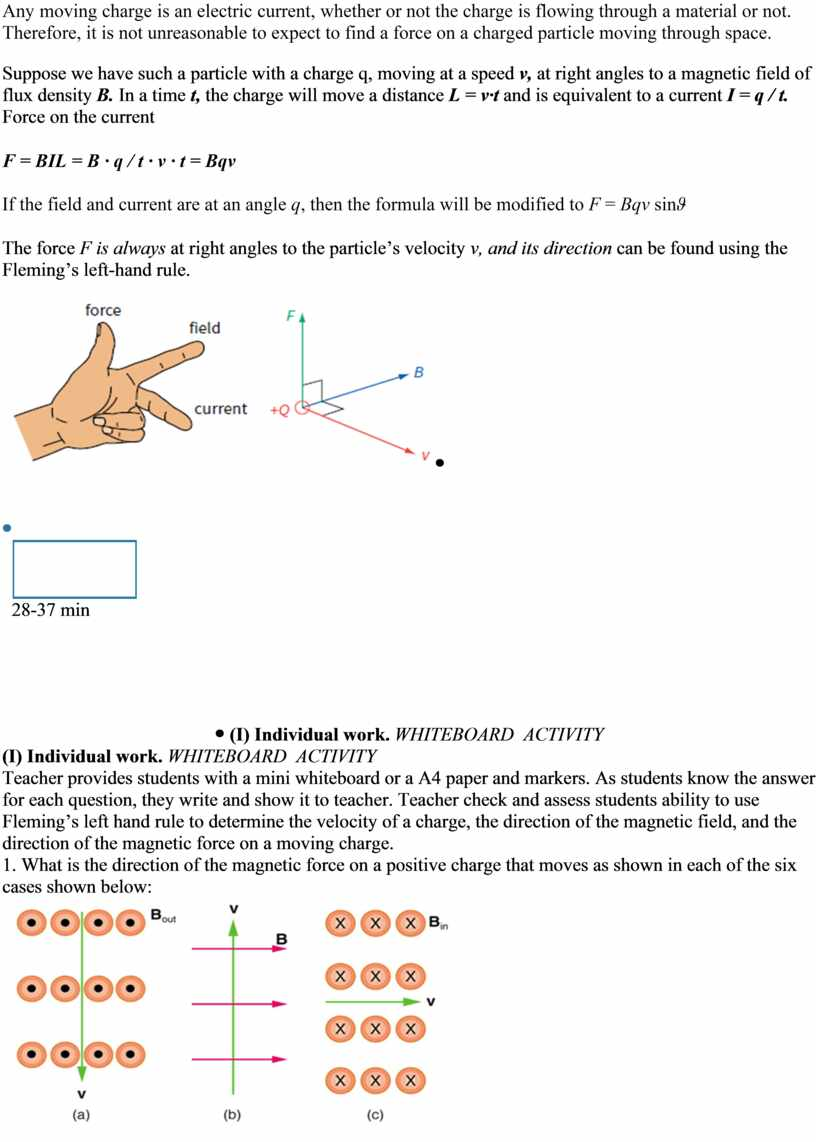## What is the maximum force on an aluminum rod with a charge that you pass between the poles of a 1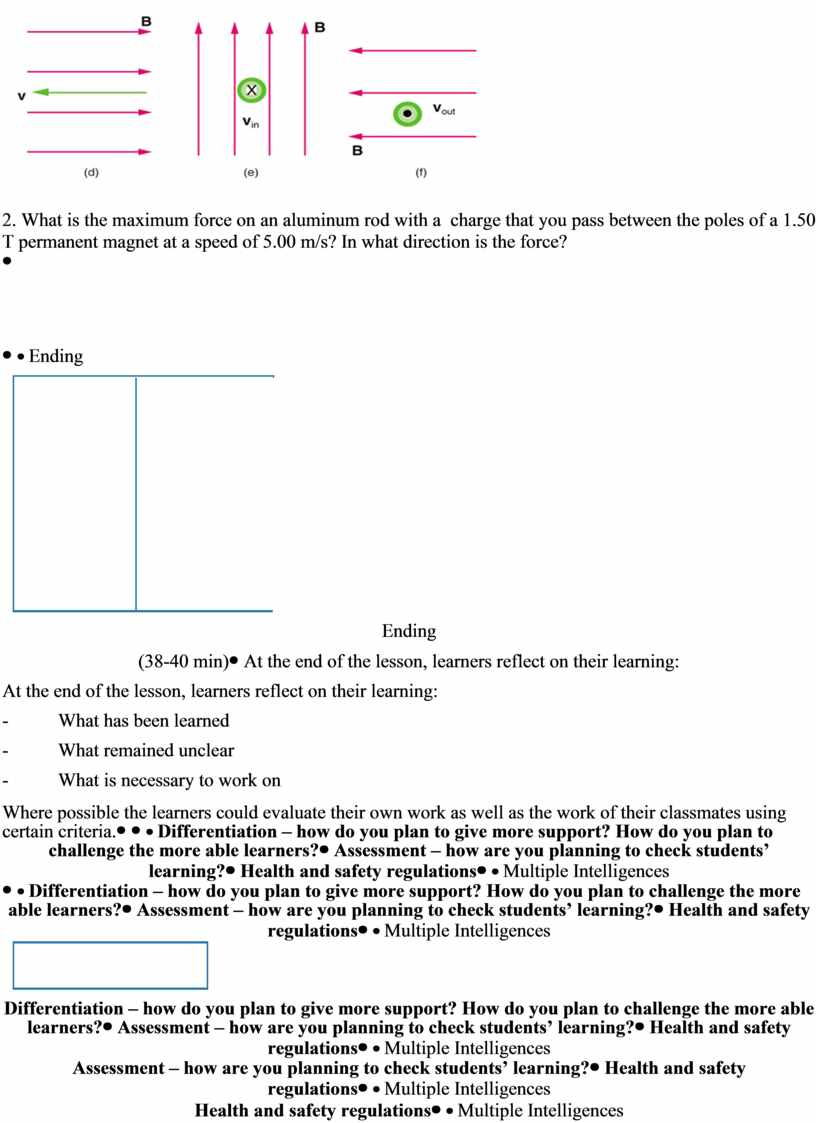Скачать файл